Courses

Ex 11.2 NCERT Solutions- Constructions Notes | Study Mathematics (Maths) Class 9 - Class 9

Class 9: Ex 11.2 NCERT Solutions- Constructions Notes | Study Mathematics (Maths) Class 9 - Class 9

The document Ex 11.2 NCERT Solutions- Constructions Notes | Study Mathematics (Maths) Class 9 - Class 9 is a part of the Class 9 Course Mathematics (Maths) Class 9.
All you need of Class 9 at this link: Class 9

Q1. Construct a triangle ABC in which BC = 7 cm, ∠B = 75º and AB + AC = 13 cm.
Ans: Steps of construction:

• Draw a ray BX.
• From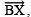cut off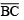= 7 cm.
• At B, construct ∠CBY = 75º.
• From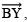cut off BD = 13 cm (= AB + BC)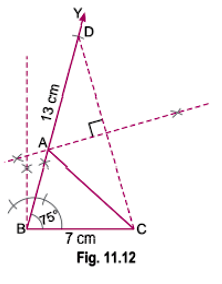• Join D and C.
• Bisect DC such that the bisector of DC meets BD at A.
• Join AC.

Thus, ΔABC is the required triangle.

Q2. Construct a triangle ABC in which BC = 8 cm, ∠ B = 45º and AB ∠ AC = 3.5 cm.
Ans: Steps of construction:

• Draw a ray• From, cut off BC = 8 cm.
• Construct ∠CBY = 45º.
• Fromcut off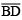= 3.5 cm.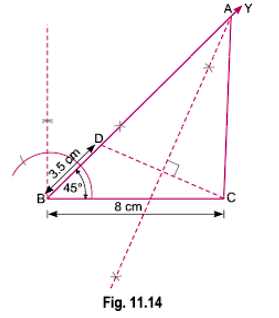• Join D and C.
• Draw PQ, the perpendicular bisector of DC, which intersectsat A.
• Join AC.

Thus, ABC is the required triangle.

Q3. Construct a triangle PQR in which QR = 6 cm, ∠Q = 60º and PR ∠ PQ = 2cm.
Ans: Steps of construction:

• Draw a ray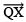.
• From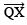, cut off QR = 6 cm.
• Construct a line YQY' such that ∠RQY = 60º.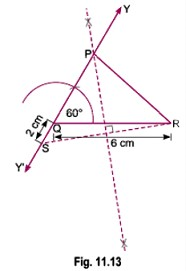• Cut off QS = 2 cm (from QY').
• Join ‘S’ and ‘R’.
• Draw MN, the perpendicular bisector of SR, which intersects QY at P.
• Join P and R.

Thus, PQR is the required triangle.

Q4. Construct a triangle XYZ in which ∠ Y = 30º , ∠ Z = 90º and XY + YZ + ZX = 11 cm.
Ans: Steps of construction: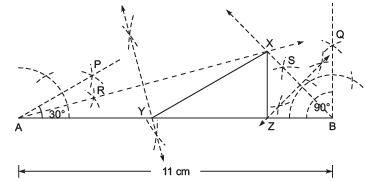• Draw a line segment AB = 11 cm = (XY + YZ + ZX)
• Construct ∠BAP = 30º = ∠Y
• Construct ∠ABQ = 90º = ∠Z
• Draw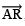, the bisector of ∠BAP.
• Draw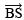, the bisector of ∠ABQ, such thatandintersect each other at X.
• Draw perpendicular bisector of AX, which intersects AB at Y.
• Draw perpendicular bisector of XB, which intersects AB at Z.
• Join XY and XZ.

Thus, XYZ is the required triangle.

Q5. Construct a right triangle whose base is 12 cm and sum of its hypotenuse and other side is 18 cm.
Ans: Steps of construction:

• Draw= 12 cm.
• Construct ∠CBY = 90º.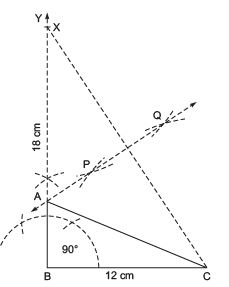• Fromcut off BX = 18 cm.
• Join CX.
• Draw PQ, the perpendicular bisector of CX, such that PQ meets BX at A.
• Join AC.

Thus, ABC is the required triangle.

The document Ex 11.2 NCERT Solutions- Constructions Notes | Study Mathematics (Maths) Class 9 - Class 9 is a part of the Class 9 Course Mathematics (Maths) Class 9.
All you need of Class 9 at this link: Class 9Use Code STAYHOME200 and get INR 200 additional OFF Use Coupon Code

Top Courses for Class 9Mathematics (Maths) Class 9

46 videos|351 docs|110 tests

Top Courses for Class 9Track your progress, build streaks, highlight & save important lessons and more!

,

,

,

,

,

,

,

,

,

,

,

,

,

,

,

,

,

,

,

,

,

;